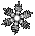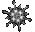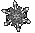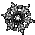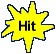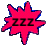Progression Puzzles - Number Sequence Patterns    Math Help Game Tips:

- The counting numbers 1,2,3,4,... form a sequence called the 'Natural' numbers = N.
The Natural numbers can also be called the 'positive Integers'.

- The sequence of numbers ...,-3,-2,-1,0,1,2,3,... is called the set of 'Integers' = I.

- A 'sequence' is a set of quantities that can be put in the same order as the positive Integers.
Each individual member of a sequence or progression is called a 'term'.

- The sequence of numbers 0,1,2,3,4,... can be called the 'Whole' number set = W. [Same as N0]

- An 'arithmetic' sequence(progression) is formed by adding a common difference to each term to get the next term.
For example the Natural numbers can be formed by using a common difference of '1'.
Another example is the arithmetic sequence 4,6,8,10,... in which the common difference is 'd=2'.

- A 'geometric' sequence(progression) is formed by multiplying each term by a common ratio to get the next term.
For example, the geometric sequence 2,6,18,54,... has the common ratio 'r=3'.

- Your Game Score is reduced by the number of snowflake hits.

- To slow the game speed repeat tap/click on the word Slider.
- To increase the game speed repeat tap/click on the word Math.
- Speed can also be adjusted with a keyboard's - and + keys.

- Refresh/Reload the web page to restart the game.